Example Questions

← Previous 1

Example Question #1 : Graphing Inequalities

For the following system of equations, what shape does the corresponding graph make: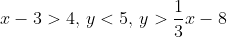Triangle

It does not form a shape.

Square

Rectangle

Triangle

Explanation:

When we graph the three inequalities as equations, we get the below graph. Testing a point within the triangle does in fact fulfill all three conditions, so the shape formed by the inequalities is a triangle. If a tested point within the triangle did not fulfill one or more conditions, then we would have chosen “it does not form a shape.”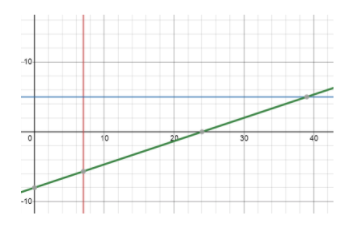Example Question #2 : Graphing Inequalities

Which of the following graphs correctly describes the system of inequalities: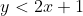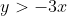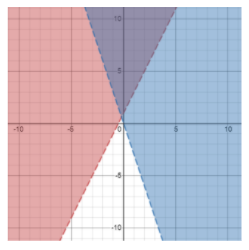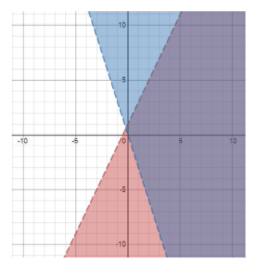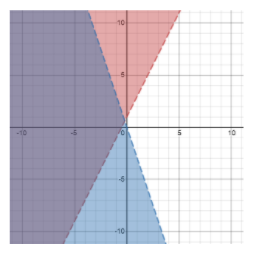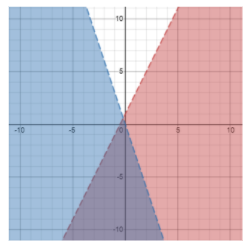Explanation:

After plotting the lines, shade the regions corresponding to each individual inequality. The area of intersection (in purple) is the solution to the system of inequalities. Another way to check your answer is to pick a point in all four regions delineated by the two equations and test those four coordinate points in the system of inequalities.

Example Question #3 : Graphing Inequalities

For the following system of equations, what shape does the corresponding graph make:Square

Rectangle

It does not form a shape

Triangle

Triangle

Explanation:

When we graph the three inequalities as equations, we get the below graph. Testing a point within the triangle does in fact fulfill all three conditions, so the shape formed by the inequalities is a triangle. If a tested point within the triangle did not fulfill one or more conditions, then we would have chosen “it does not form a shape.”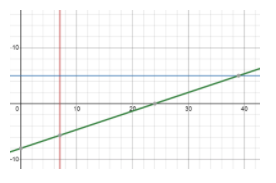Example Question #4 : Graphing Inequalities

Which of the following graphs correctly describes the system of inequalities: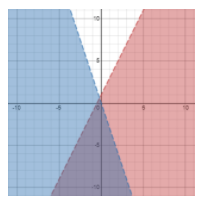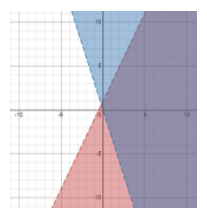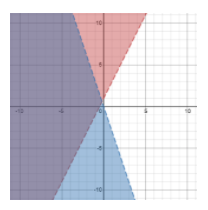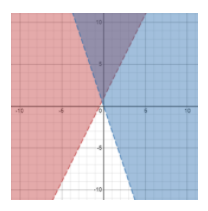Explanation:

After plotting the lines, shade the regions corresponding to each individual inequality. The area of intersection (in purple) is the solution to the system of inequalities. Another way to check your answer is to pick a point in all four regions delineated by the two equations and test those four coordinate points in the system of inequalities.

Example Question #5 : Graphing Inequalities

Which of the following ordered pairs (x,y) is a solution to the system of inequalities: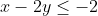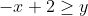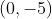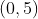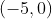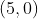Explanation:

This is the only coordinate point that fulfills the system of inequalities.

Example Question #6 : Graphing Inequalities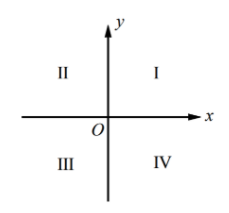If the system of inequalities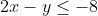and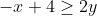is graphed on the xy- plane above, which quadrant contains no solutions to the system?

III

I and II

I

II

I and II

Explanation:

The graph of the system of inequalities is shown below. The solution (in purple) does not go into region I or II.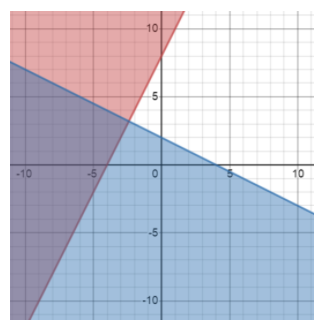Example Question #7 : Graphing Inequalities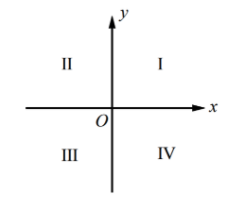If the system of inequalities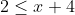andis graphed on the xy- plane above, which quadrant contains no solutions to the system?

III

IV

There are solutions in all 4 quadrants.

II

There are solutions in all 4 quadrants.

Explanation:

The graph of the system of inequalities is shown below. The solution (in purple) is present in all four regions.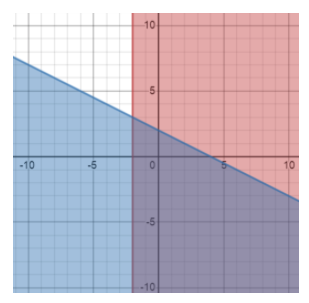Example Question #8 : Graphing Inequalities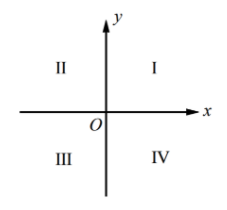If the system of inequalities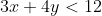and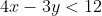is graphed on the xy- plane above, which quadrant contains no solutions to the system?

IV

III

II

There are solutions in all 4 quadrants.

There are solutions in all 4 quadrants.

Explanation:

The graph of the system of inequalities is shown below. The solution (in purple) is present in all four regions.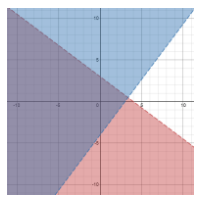Example Question #9 : Graphing Inequalities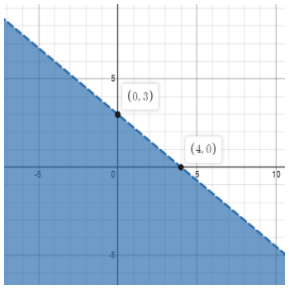The graph shown above is best represented by which inequality?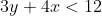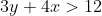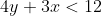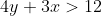Explanation:

The x-intercept is (4,0) so when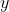equals 0,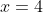, and the y-intercept is (0,3) so when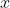equals 0,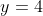. These points only work for a line corresponding to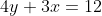. To determine which direction the inequality points, you can plug in any point. If we use (0,0),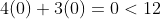, so this is the direction the inequality must face.

Example Question #10 : Graphing Inequalities

Which of the following are solutions to the system of equations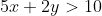and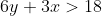?

I)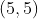II)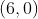III)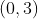II

I and II

I

I, II, and III

With this question, it is important to recognize the system of inequalities utilizes the “greater than” sign, not the “greater than or equal to” sign.does not fulfillappears to fulfill both conditions but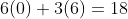. 18 is not greater than 8, leaving onlyas a possible solution.